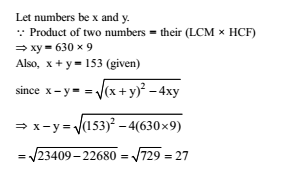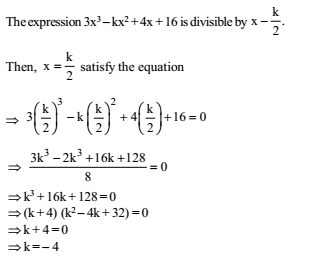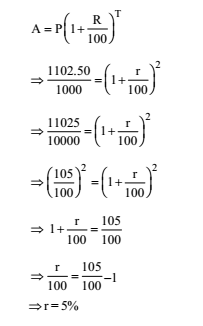# RRB ALP 2018 Practice Test Papers | Arithmetic Questions (Day-43)

Dear Aspirants, Here we have given the Important RRB ALP & Technicians Exam 2018 Practice Test Papers. Candidates those who are preparing for RRB ALP 2018 can practice these Arithmetic Questions to get more confidence to Crack RRB 2018 Examination.

[WpProQuiz 2217]

Click “Start Quiz” to attend these Questions and view Explanation

1. Nitin’s age was equal to square of some number last year and the following year it would be cube of a number. If again Nitin’s age has to be equal to the cube of some number, then for how long he will have to wait?

(a) 10 years

(b) 38 years

(c) 39 years

(d) 64 years

1. A bag contains Rs 216 in the form of one rupee, 50 paise and 25 paise coins in the ratio of 2: 3: 4. The number of 50 paise coins is:

(a) 96

(b) 144

(c) 114

(d) 141

1. A started a business with Rs. 4500 and another person B joined after some period with Rs. 3000. Determine this period after B joined the business if the profit at the end of the year is divided in the ratio 2: 1

(a) After 3 months

(b) After 4 months

(c) After 6 months

(d) After 2  1/2 months

1. The L.C.M. of two number is 630 and their H.C.F. is 9. If the sum of numbers is 153, their difference is

(a) 17

(b) 23

(c) 27

(d) 33

1. If a dividend of Rs. 57,834 is to be divided among Meena, Urmila and Vaishali in the proportion of 3:2:1, find Urmila’s share.

(a) Rs.  19,281

(b) Rs.  17,350

(c) Rs.  23,133

(d) Rs.  19,278

1. The average age of a man and his son is 16 years. The ratio of their ages is 15: 1 respectively. What is the son’s age?

(a) 30 years

(b) 32 years

(c) 2 years

(d) 4 years

1. If Rs. 1000 is divided between A and B in the ratio 3: 2, then A will receive

(a) Rs. 400

(b) Rs. 500

(c) Rs. 600

(d) Rs. 800

1. A manufacturer marked an article at Rs. 50 and sold it allowing 20% discount. If his profit was 25% then the cost price of the article was

(a) Rs. 40

(b) Rs. 35

(c) Rs. 32

(d) Rs. 30

1. For what value of k, will the expression 3x3 – kx2 + 4x + 16 be divisible by x-k/2 ?

(a) 4

(b) –4

(c) 2

(d) 0

1. At what rate per cent per annum will a sum of Rs. 1,000 amount to Rs. 1,102.50 in 2 years at compound interest?

(a) 5

(b) 5.5

(c) 6

(d) 6.5

Clearly, we have to first find two numbers whose difference is 2 and of which the smaller one is a perfect square and the bigger one a perfect cube. Such numbers are 25 and 27. Thus, Nitin is now 26 years old. Since the next perfect cube after 27 is 64, So required time period = (64 – 26) years = 38 years.

Let the no. of one rupee, 50 paise and 25 paise coins be 2x, 3x and 4x respectively.

According to question,

2x+3x/2+4x/4 =Rs. 216

(8x+6x+4x)/4 =216

x = 48

Number of 50 paise coins = 48 × 3 = 144

Let B joined after x months.

Then, 4500 × 12: 3000 (12 – x) = 2: 1

Ratio of their investments

4500×12/(3000(12-x))=2/1Share of Urmila in dividend = 2/6 x 57834  =  19278

Let the age of father and son be 15x years and x years respectively.

Now, according to the question, 15x+ x /2 = 16 or, x= 16×2/16 =2 years

Hence age of the son = 2 years

A’s share= 3/5×1000 =600

Marked price = Rs. 50

S.P. after discount = 80% of 50 = Rs. 40

If the CP of article be Rs. x, then 125 * x/100  = 40

x= Rs. 32